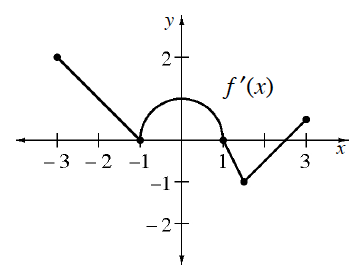### Home > CALC3RD > Chapter Ch5 > Lesson 5.4.1 > Problem5-142

5-142.

Given $f(t)=2+\int_0^tf^\prime(x)dx$, where $y = f ′(x)$ is shown on the graph in problem 5-141. Calculate the following values:

1. $f (1)$

This is $2$ plus the area under the curve for $0 ≤ x ≤ 1$.2. $f ^\prime(1)$

Notice that the graph is of $f '\left(x\right)$.

3. $f (-3)$

Refer to hint in part (a).

4. $f^\prime(-2)$

Refer to hint in part (b).

5. $f ^{\prime \prime}(2)$

$f ''\left(x\right)$ represents the slope of $f '\left(x\right)$ and you are looking at the $f '\left(x\right)$ graph.